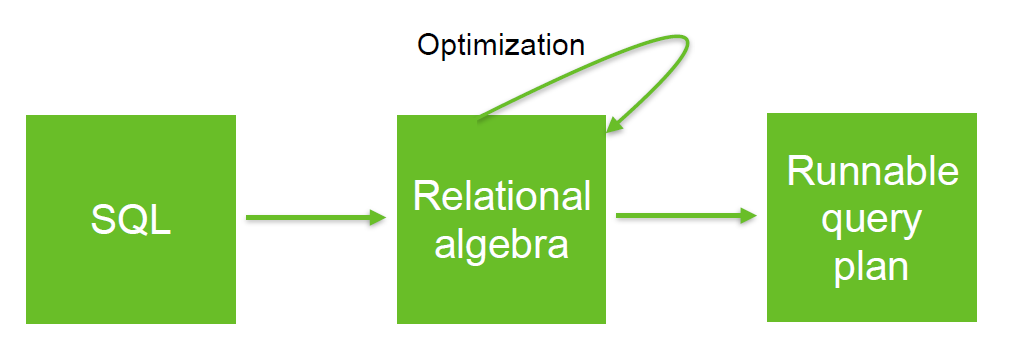# Nested Loop - Adding More Tables

When a nested loops join uses four tables, the optimizer performs the following steps:

1. Select a driving table.
2. Perform a NESTED LOOPS join between the driving table and the second table.
3. Perform a NESTED LOOPS join between the driving set returned from step 2 and the third table.
4. Perform a NESTED LOOPS join between the driving set returned from step 3 and fourth table.

When an improper driving table is used, the first NESTED LOOPS join will perform poorly. However, selecting the proper driving table does not guarantee good performance of a join that uses two or more tables. When there are more than two tables, the size of the driving set of records involved in each successive join must be considered. When the driving set is not selective, the performance of each successive join will decrease.

Adding tables to the NESTED LOOPS increases the time needed to complete the query exponentially!

When the column used to join tables is based on a limiting condition in a WHERE clause, use the table to which the condition is applied.

For example, with four tables (named A, B, C, and D) in a query, all of which are the same size and use the following FROM and WHERE clauses:

``````from D, C, B, A
where A.join_column = B.join_column
and B.join_column = C.join_column
and C.join_column = D.join_column
and A.join_column = 12345
and D.Name = ‘MAGELLAN’```
```

A NESTED LOOPS join can be used in this example when A, B, C, and D have indexes on their join columns. This results in the following:

1. Table A is joined to table B (AB).
2. The driving set from AB is joined to table C (ABC).
3. The driving set from ABC is joined to table D, and the limiting condition on D.Name is applied.

When D.Name is selective, the performance of the query can be improved by joining D as part of the first NESTED LOOPS join in the execution path. This improves performance because the first NESTED LOOPS join returns fewer records. A smaller driving set is then used for each successive join.

Just as a large driving set can cause significant performance degradation, using a small driving set can yield significant performance improvement.

In the following example, the WHERE clause is rewritten, moving the limiting condition from A to D instead:

``````from D, C, B, A
where A.join_column = B.join_column
and B.join_column = C.join_column
and C.join_column = D.join_column
and D.join_column = 12345
and D.Name = ‘MAGELLAN’```
```

Using this revised WHERE clause, the optimizer performs the following joins:

1. Table D is joined to table C (DC).
2. The driving set from DC is joined to table B (DCB).
3. The driving set from DCB is joined to table A.

This revised WHERE clause significantly improves the query performance.

For example, tables A, B, C, and D have exactly 100 records, and there is only one record in D with a Name value equal to MAGELLAN. The number of buffer gets (logical reads) required to resolve the original query is shown in the following table.

An Oracle Database - (B|Balanced) Tree - BTree indexes access generates an average of 2 accesses inside the index “branches”, plus 1 access to the index “leaf” blocks for each row and 1 ROWID access to the table for each row.

Accessing 100 rows by an index search requires 2 buffer gets for the index branches, plus 100 index reads and 100 table reads, for a total of 202 reads. For the join from A to B, 100 buffer gets are required for each of the 202 buffer gets required by the read of table A.

Cumulative Buffer Gets Required by the Original NESTED LOOPS Join

Operation Table Accessed Buffer Gets Cumulative Buffer Gets
First Table Accessed A 2+2*100 202
First Joined Table B 100*202 20,402
Second Joined Table C 100*100*202 2,040,402
Third Joined Table D 100*100*100*202 204,040,402

As shown in this table, each successive join procedure generates excessive buffer gets when the driving set from the preceding join is large. The following table shows the number of buffer gets that are used when the revised WHERE clause is used (in which the limiting condition on the join column was moved to table D, which had an additional limiting condition).

Cumulative Buffer Gets for Modified NESTED LOOPS Join

Operation Table Accessed Buffer Gets Cumulative Buffer Gets
First Table Accessed D 2+2*100 202
First Joined Table C 1*202 404
Second Joined Table B 100*1*202 20,604
Third Joined Table A 100*100*1*202 2,040,604

The revised WHERE clause made a significant difference to the number of buffer gets that this database needed to perform the query. In the original query, the limiting conditions on table D did not affect the size of the driving sets, because it was the last table that was joined in the query. In the revised query, table D is part of the first NESTED LOOPS join, and now the query is completed only using one one-hundredth of the buffer gets as the original query. The modified query runs significantly faster than the original query.

Recommended PagesRelation Operator - Nested Loop Join

Nested loop joins are join operator. They are useful when small subsets of data are being joined and if the join condition is an efficient way of accessing the second table. NESTED LOOPS is a row operation....